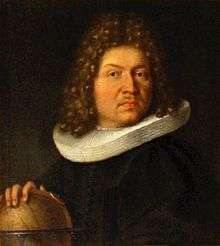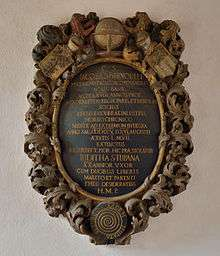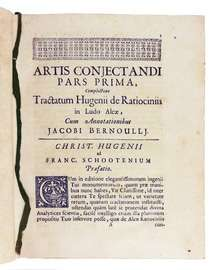# Jacob Bernoulli

For other family members named Jacob, see Bernoulli family.
Jacob BernoulliJacob Bernoulli
Born 27 December 1654
Basel, Switzerland
Died 16 August 1705 (aged 50)
Basel, Switzerland
Residence Switzerland
Fields Mathematics, mechanics
Institutions University of Basel
Alma mater University of Basel
(D.Th., 1676; Dr.phil.hab., 1684)
Theses
Doctoral students Johann Bernoulli
Jacob Hermann
Nicolaus I Bernoulli
Known for Bernoulli differential equation
Bernoulli numbers
Bernoulli's formula
Bernoulli polynomials
Bernoulli map
Bernoulli trial
Bernoulli process
Bernoulli scheme
Bernoulli operator
Hidden Bernoulli model
Bernoulli sampling
Bernoulli distribution
Bernoulli random variable
Bernoulli's Golden Theorem
Bernoulli's inequality
Lemniscate of Bernoulli
Influences Nicolas Malebranche
Notes
Brother of Johann Bernoulli

Jacob Bernoulli (also known as James or Jacques; 6 January 1655 [O.S. 27 December 1654] – 16 August 1705) was one of the many prominent mathematicians in the Bernoulli family. He was an early proponent of Leibnizian calculus and had sided with Leibniz during the Leibniz–Newton calculus controversy. He is known for his numerous contributions to calculus, and along with his brother Johann, was one of the founders of the calculus of variations. However, his most important contribution was in the field of probability, where he derived the first version of the law of large numbers in his work Ars Conjectandi.

## Biography

Jacob Bernoulli was born in Basel, Switzerland. Following his father's wish, he studied theology and entered the ministry. But contrary to the desires of his parents, he also studied mathematics and astronomy. He traveled throughout Europe from 1676 to 1682, learning about the latest discoveries in mathematics and the sciences under leading figures of the time. This included the work of Johannes Hudde, Robert Boyle, and Robert Hooke. During this time he also produced an incorrect theory of comets.

Bernoulli returned to Switzerland and began teaching mechanics at the University in Basel from 1683. In 1684 he married Judith Stupanus; and they had two children. During this decade, he also began a fertile research career. His travels allowed him to establish correspondence with many leading mathematicians and scientists of his era, which he maintained throughout his life. During this time, he studied the new discoveries in mathematics, including Christiaan Huygens's De ratiociniis in aleae ludo, Descartes' Geometrie and Frans van Schooten's supplements of it. He also studied Isaac Barrow and John Wallis, leading to his interest in infinitesimal geometry. Apart from these, it was between 1684 and 1689 that many of the results that were to make up Ars Conjectandi were discovered.

He was appointed professor of mathematics at the University of Basel in 1687, remaining in this position for the rest of his life. By that time, he had begun tutoring his brother Johann Bernoulli on mathematical topics. The two brothers began to study the calculus as presented by Leibniz in his 1684 paper on the differential calculus in "Nova Methodus pro Maximis et Minimis" published in Acta Eruditorum. They also studied the publications of von Tschirnhaus. It must be understood that Leibniz's publications on the calculus were very obscure to mathematicians of that time and the Bernoullis were the first to try to understand and apply Leibniz's theories.

Jacob collaborated with his brother on various applications of calculus. However the atmosphere of collaboration between the two brothers turned into rivalry as Johann's own mathematical genius began to mature, with both of them attacking each other in print, and posing difficult mathematical challenges to test each other's skills. By 1697 the relationship had completely broken down.

Jacob Bernoulli died in 1705. Bernoulli chose a figure of a logarithmic spiral and the motto Eadem mutata resurgo ("Changed and yet the same, I rise again") for his gravestone; the spiral executed by the stonemasons was, however, an Archimedean spiral, “[Jacob Bernoulli] wrote that the logarithmic spiral ‘may be used as a symbol, either of fortitude and constancy in adversity, or of the human body, which after all its changes, even after death, will be restored to its exact and perfect self’.” (Livio 2002: 116). His grave is in Basel Munster or Cathedral where the gravestone shown below is located.

The lunar crater Bernoulli is also named after him jointly with his brother Johann.

## Important worksJacob Bernoulli's grave.

Jacob Bernoulli's first important contributions were a pamphlet on the parallels of logic and algebra published in 1685, work on probability in 1685 and geometry in 1687. His geometry result gave a construction to divide any triangle into four equal parts with two perpendicular lines.

By 1689 he had published important work on infinite series and published his law of large numbers in probability theory. Jacob Bernoulli published five treatises on infinite series between 1682 and 1704 The first two of these contained many results, such as the fundamental result thatdiverges, which Bernoulli believed were new but they had actually been proved by Mengoli 40 years earlier. Bernoulli could not find a closed form for, but he did show that it converged to a finite limit less than 2. Euler was the first to find the sum of this series in 1737. Bernoulli also studied the exponential series which came out of examining compound interest.

In May 1690 in a paper published in Acta Eruditorum, Jacob Bernoulli showed that the problem of determining the isochrone is equivalent to solving a first-order nonlinear differential equation. The isochrone, or curve of constant descent, is the curve along which a particle will descend under gravity from any point to the bottom in exactly the same time, no matter what the starting point. It had been studied by Huygens in 1687 and Leibniz in 1689. After finding the differential equation, Bernoulli then solved it by what we now call separation of variables. Jacob Bernoulli's paper of 1690 is important for the history of calculus, since the term integral appears for the first time with its integration meaning. In 1696 Bernoulli solved the equation, now called the Bernoulli differential equation,Jacob Bernoulli also discovered a general method to determine evolutes of a curve as the envelope of its circles of curvature. He also investigated caustic curves and in particular he studied these associated curves of the parabola, the logarithmic spiral and epicycloids around 1692. The lemniscate of Bernoulli was first conceived by Jacob Bernoulli in 1694. In 1695 he investigated the drawbridge problem which seeks the curve required so that a weight sliding along the cable always keeps the drawbridge balanced.

Jacob Bernoulli's most original work was Ars Conjectandi published in Basel in 1713, eight years after his death. The work was incomplete at the time of his death but it is still a work of the greatest significance in the theory of probability. In the book Bernoulli reviewed work of others on probability, in particular work by van Schooten, Leibniz, and Prestet. The Bernoulli numbers appear in the book in a discussion of the exponential series. Many examples are given on how much one would expect to win playing various games of chance. The term Bernoulli trial resulted from this work. There are interesting thoughts on what probability really is:

... probability as a measurable degree of certainty; necessity and chance; moral versus mathematical expectation; a priori an a posteriori probability; expectation of winning when players are divided according to dexterity; regard of all available arguments, their valuation, and their calculable evaluation; law of large numbers ...

Bernoulli was one of the most significant promoters of the formal methods of higher analysis. Astuteness and elegance are seldom found in his method of presentation and expression, but there is a maximum of integrity.

## Discovery of the mathematical constant eArs conjectandi, 1713 (Milano, Fondazione Mansutti).

in 1683 Bernoulli discovered the constant e by studying a question about compound interest which required him to find the value of the following expression (which is in fact e):One example is an account that starts with $1.00 and pays 100 percent interest per year. If the interest is credited once, at the end of the year, the value is$2.00; but if the interest is computed and added twice in the year, the $1 is multiplied by 1.5 twice, yielding$1.00×1.5² = $2.25. Compounding quarterly yields$1.00×1.254 = $2.4414..., and compounding monthly yields$1.00×(1.0833...)12 = $2.613035.... Bernoulli noticed that this sequence approaches a limit (the force of interest) for more and smaller compounding intervals. Compounding weekly yields$2.692597..., while compounding daily yields $2.714567..., just two cents more. Using n as the number of compounding intervals, with interest of 100%/n in each interval, the limit for large n is the number that Euler later named e; with continuous compounding, the account value will reach$2.7182818.... More generally, an account that starts at \$1, and yields (1+R) dollars at simple interest, will yield eR dollars with continuous compounding.

## Translation of Latin inscription on Bernoulli's tomb

IACOBUS BERNOULLI
MATHEMATICUS INCOMPARABILIS
VLTRA XVIII ANNOS PROF.
ACADEM. ITEM REGIAE PARIS. ET BEROLIN.
SOCIUS
EDITIS LUCUBRAT. INLUSTRIS.
MORBO CHRONICO
ANNO SALUT. MDCCV. D. XVI. AUGUSTI
AETATIS L. M. VII
EXTINCTUS
RESURRECT. PIOR. HIC PRAESTOLATUR
IUDITHA STUPANA
XX ANNOR. UXOR
CUM DUOBUS LIBERIS
MARITO ET PARENTI
EHEU DESIDERATISS.
H.M.P.
Jacob Bernoulli, the incomparable mathematician.
Professor at the University of Basel For more than 18 years;
member of the Royal Academies of Paris and Berlin; famous for his writings.
Of a chronic illness, of sound mind to the end;
succumbed in the year of grace 1705, the 16th of August, at the age of 50 years and 7 months, awaiting the resurrection.
Judith Stupanus,
his wife for 20 years,
and his two children have erected a monument to the husband and father they miss so much.
1. Jacob (Jacques) Bernoulli, The MacTutor History of Mathematics archive, School of Mathematics and Statistics, University of St Andrews, UK.
2. Nagel, Fritz (11 June 2004). "Bernoulli, Jacob". Historisches Lexikon der Schweiz. Retrieved 20 May 2016.
3. Pfeiffer, Jeanne (November 2006). "Jacob Bernoulli" (PDF). Journ@l électronique d'Histoire des Probabilités et de la Statistique. Retrieved 20 May 2016.
4. Jacob Bernoulli (1690) "Quæstiones nonnullæ de usuris, cum solutione problematis de sorte alearum, propositi in Ephem. Gall. A. 1685" (Some questions about interest, with a solution of a problem about games of chance, proposed in the Journal des Savants (Ephemerides Eruditorum Gallicanæ), in the year (anno) 1685.**), Acta eruditorum, pp. 219–23. On p. 222, Bernoulli poses the question: "Alterius naturæ hoc Problema est: Quæritur, si creditor aliquis pecuniæ summam fænori exponat, ea lege, ut singulis momentis pars proportionalis usuræ annuæ sorti annumeretur; quantum ipsi finito anno debeatur?" (This is a problem of another kind: The question is, if some lender were to invest [a] sum of money [at] interest, let it accumulate, so that [at] every moment [it] were to receive [a] proportional part of [its] annual interest; how much would he be owed [at the] end of [the] year?) Bernoulli constructs a power series to calculate the answer, and then writes: " … quæ nostra serie [mathematical expression for a geometric series] &c. major est. … si a=b, debebitur plu quam 2½a & minus quam 3a." ( … which our series [a geometric series] is larger [than]. … if a=b, [the lender] will be owed more than 2½a and less than 3a.) If a=b, the geometric series reduces to the series for a × e, so 2.5 < e < 3. (** The reference is to a problem which Jacob Bernoulli posed and which appears in the Journal des Sçavans of 1685 at the bottom of page 314.)
5. J J O'Connor and E F Robertson. "The number e". St Andrews University. Retrieved 2 November 2016.